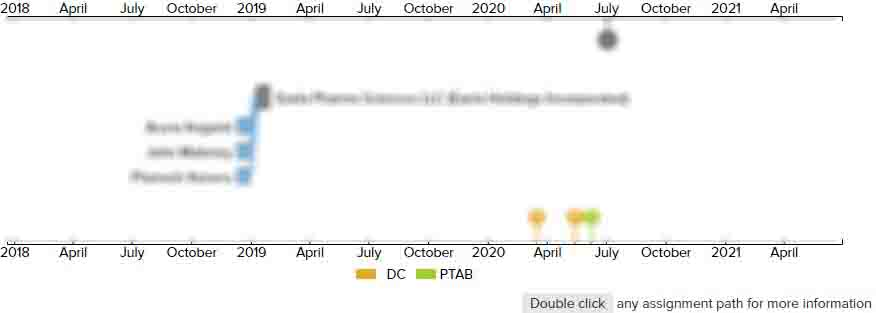# Selection device

• US 9,735,796 B2
• Filed: 10/15/2015
• Issued: 08/15/2017
• Est. Priority Date: 12/09/2009
• Status: Active Grant
##### First Claim
Patent Images

1. A selection device receiving a digital signals and outputting a total value of outputs of a plurality of unit cells being a drive circuit which drives a voice coil, each of the unit cells outputting a plus value and a minus value, comprising:

• a circuit outputting a signal representing a number of unit cells outputting a plus value among the plurality of unit cells, and a signal representing a number of unit cells outputting a minus value among the plurality of unit cells;

a selection circuit of a plus side selecting a unit cell outputting a plus value among the plurality of unit cells based on a frequency of selection of the unit cell, wherein a number of unit cells outputting a plus value and selected by the selection circuit of a plus side is determined by the signal representing a number of unit cells outputting a plus value among the plurality of unit cells,a selection circuit of a minus side selecting a unit cell outputting a minus value from the plurality of unit cells based on a frequency of selection of the unit cell, wherein a number of unit cells outputting a minus value and selected by the selection circuit of a minus side determined by the signal representing a number of cells outputting a minus value among the plurality of unit cells,wherein, taking the average in time of the summation of the plus value unit cell and the minus value unit cell will represent the digital signal value.

• ##### 2 Assignments
Timeline View
Assignment View×
×